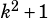For an real number k, which of the following must be a real number?

A)1/k
B)k/k+1
C)1/k-1
D) k/k^2+1
E) 1/k^2-1

Thank you

If it’s possible to make the denominator equal zero, then you can eliminate the choice. (A) is not real when k = 0. (B) is not real when k = -1. (C) is not real when k = 1. (E) is not real when k = 1 or -1.

(D) is the answer because there’s no value of k that makesequal zero.